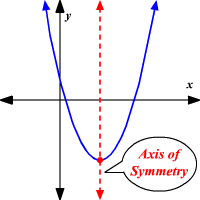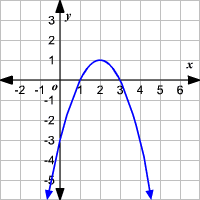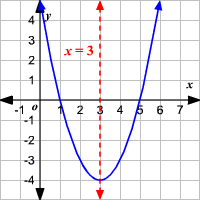# Axis of Symmetry of a Parabola

The graph of a quadratic function is a parabola. The axis of symmetry of a parabola is a vertical line that divides the parabola into two congruent halves. The axis of symmetry always passes through the vertex of the parabola . The $x$ -coordinate of the vertex is the equation of the axis of symmetry of the parabola.For a quadratic function in standard form, $y=a{x}^{2}+bx+c$ , the axis of symmetry is a vertical line $x=-\frac{b}{2a}$ .

Example 1:

Find the axis of symmetry of the parabola shown.The $x$ -coordinate of the vertex is the equation of the axis of symmetry of the parabola.

The vertex of the parabola is $\left(2,1\right)$ .

So, the axis of symmetry is the line $x=2$ .

Example 2:

Find the axis of symmetry of the graph of $y={x}^{2}-6x+5$ using the formula.

For a quadratic function in standard form, $y=a{x}^{2}+bx+c$ , the axis of symmetry is a vertical line $x=-\frac{b}{2a}$ .

Here, $a=1,b=-6$ and $c=5$ .

Substitute.

$x=-\frac{-6}{2\left(1\right)}$

Simplify.

$\begin{array}{l}x=\frac{6}{2}\\ \text{\hspace{0.17em}}\text{\hspace{0.17em}}\text{\hspace{0.17em}}\text{\hspace{0.17em}}=3\end{array}$

Therefore, the axis of symmetry is $x=3$ .